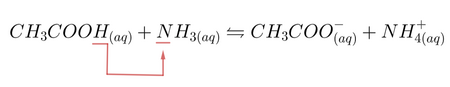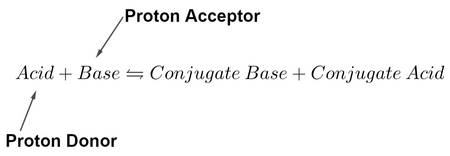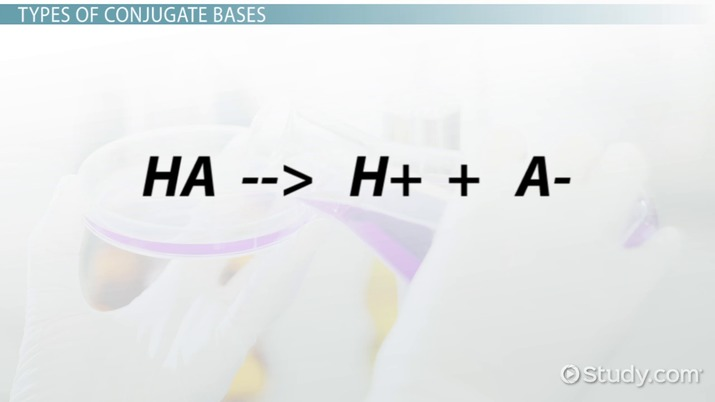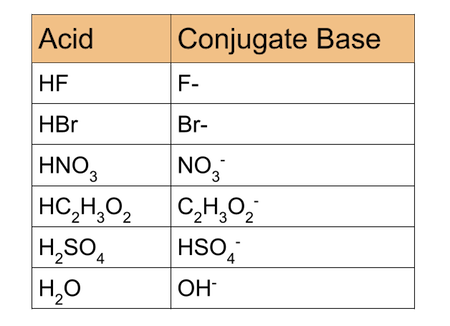Science Courses / Course / Chapter

# Conjugate Base Overview & Examples

• Author

A freelance tutor currently pursuing a master's of science in chemical engineering. Graduated from the American University of the Middle East with a GPA of 3.87, performed a number of scientific primary and secondary research. Tutored university level students in various courses in chemical engineering, math, and art. Has experience tutoring middle school and high school level students in science courses.

• Instructor
Elizabeth (Nikki) Wyman

Nikki has a master's degree in teaching chemistry and has taught high school chemistry, biology and astronomy.

Define conjugate base in chemistry. Learn how to find the conjugate base and see examples of conjugate bases in common chemistry problems. Updated: 01/09/2022

Show

## What is a Conjugate Base?

The conjugation definition chemistry refers to a species ability to form a link with another species. The conjugate base definition describes a chemical compound that was originally an acid that has lost its proton. The conjugate base is an ex-acid that lost its positively charged hydrogen when reacting with a proton acceptor. This chemical compound has the ability to accept a proton to, once again, transform back into an acid. The reason why these compounds are called conjugate bases is because:

• They act as bases by accepting protons.
• They have the ability to reform the lost link with their protons. The word conjugation means linking with other species.

An acid is a substance that donates at least one proton to another substance. Once it does that, it will turn into a conjugate base. Taking hydrofluoric acid {eq}HF {/eq} as example. Weak as it may be, it is still capable of losing its proton to form the conjugate base {eq}F^- {/eq}, as shown in its dissociation equation:

{eq}HF \leftrightharpoons H^+ + F^- {/eq}

Hydrofluoric acid dissociates into its respective conjugate base {eq}F^- {/eq} and to protons {eq}H^+ {/eq}. The conjugate base {eq}F^- {/eq} behave as most bases behave; by accepting protons from other atoms. The table serves as a brief overview of acids, bases, conjugate acids, and bases. Conjugate acids are the particles formed after its base accepts a proton.

Parameter Acid Base
Defined as Proton donors Proton acceptors
Dissociates into Protons and the conjugate base Hydroxyl ions and conjugate acid
pH pH of acids > 7 pH of bases < 7
Taste Sour Soapy, alkaline-like
Litmus Paper Reaction Turn blue litmus paper to red Turn red litmus paper to blue
Parameter Conjugate Base Conjugate Acid
Behavior Behaves like bases by accepting protons Behaves like acids by donating protons
Formation Formed when the acid loses its proton Formed when the base accepts protons

It should be noted that protons {eq}H^+ {/eq} never existed freely in aqueous solutions; there is no such solution that is composed of nothing but protons. Protons are always within some electron density.

### Bronsted-Lowry Acid-Base Theory

Bronsted-Lowry Acid-Base Theory defines acids and bases as:

• Compounds that give up their protons are acids.
• Compounds that accept protons are bases.

The term conjugate base comes from the Bronsted-Lowry Acid-Base Theory because they originate from acids, but they act as bases. They act as bases by having the tendency to accept protons. A conjugate acid acts like most acids do, by donating protons. Conjugate acids are originally bases that accepted protons from different molecules. Conjugate bases and conjugate acids are formed in acid-base reactions, where an actual acid reacts with an actual base. An example of an acid-base reaction is the following, the reaction of acetic acid with the base, ammonia.Where:

• Acetic acid {eq}CH_3COOH {/eq} is the acid. It donates its proton to the base.
• Ammonia {eq}NH_3 {/eq} is the base. It accepts a proton from the acid.
• Acetate anion {eq}CH_3COO^- {/eq} is the conjugate base. It is formed when the acetic acid loses its proton. The acetate anion is capable of accepting a proton and becoming an acid again.
• Ammonium ion {eq}NH^+_4 {/eq} is the conjugate acid. It is formed when ammonia gains an additional proton. The ammonium ion is capable of donating its new proton and reverting back to a base.

It can be concluded that in acid-base reactions, the acid transfers its proton to the base to form their respective conjugate base and conjugate acid. The general equation between acids and bases that are in equilibrium with one another is shown by the following.### The Strength of Conjugate Bases

The strength of the conjugate base can be predicated by having knowledge about its acid. As the old saying in chemistry goes, the conjugate base of a strong acid is always weak. According to Brostond-Lowry's theory, the strength of an acid is assessed by its ability to donate its proton(s). Strong acids readily give up their protons; their attachment to their {eq}H^+ {/eq} is weak, which is why they have no quarrel in giving them up. Weak acids are the opposite. Their attachment to their protons is so strong that they do not give them up easily. The equilibrium constant, Ka, for acids is a measure of how strong an acid is and how well it dissociates into its respective ions.

• Strong acids dissociate completely into their conjugate bases and protons. Their Ka value is high.
• Weak acids dissociate partially into their conjugate bases and protons. Their Ka value is low.

The following can be deduced about the strength of conjugate bases.

• The conjugate base of a strong acid is weak. Its tendency to reaccept its lost proton is weak.
• The conjugate base of a weak acid is strong. Its tendency to reaccept its lost proton is strong.

HCl is a strong acid, its conjugate base is weak. Hydrochloric acid dissociates completely into its ions. There is no equilibrium, which is why its conjugate base, {eq}Cl^- {/eq}, does not reattach itself to a proton.

{eq}HCl_{(aq)} \rightarrow H^+_{(aq)} + Cl^-_{(aq)} {/eq}

Acetic acid, on the other hand, is a very weak acid. Its conjugate base is strong. The acetate ion {eq}CH_3COO^- {/eq} is capable of restoring its bond with its lost proton. This explains why the dissociation reactions is at equilibrium.

{eq}CH_3COOH_{(aq)} \leftrightharpoons H^+_{(aq)} + CH_3COO^-_{(aq)} {/eq}

The table documents a few of the important acids along with their respective conjugate bases. The strength of the acid decreases going down the column, while the strength of the conjugate base increases.

## Conjugate Bases

If we approach conjugate bases like experienced detectives, the first place to investigate them is the last place we would expect them to be: in the world of acids. Chemically speaking, acids are the opposite of bases. In general, acids are substances that break apart in water to produce hydrogen ions (H+). They have low pH values, taste, and smell sour, and can be corrosive.

Conjugate bases are intimately related to acids. A conjugate base is the particle that is left over after the acid loses its hydrogen ion. Most of the time, conjugate bases are negatively charged and have high pH values.An error occurred trying to load this video.

Try refreshing the page, or contact customer support.

Coming up next: Denaturation of Protein: Definition & Causes

### You're on a roll. Keep up the good work!

Replay
Your next lesson will play in 10 seconds
• 0:01 Conjugate Bases
• 0:45 Types of Conjugate Bases
• 2:23 Acid-Base Reactions
• 3:10 Lesson Sumary
Save Save

Want to watch this again later?

Timeline
Autoplay
Autoplay
Speed Speed

## Types of Conjugate Bases

There are as many conjugate bases as there are acids. When an acid loses a hydrogen ion, its conjugate base is produced. An acid and its conjugate base have the same formula but with one minor difference: an acid has one more hydrogen ion than the conjugate base does.

Let's take hydrochloric acid (HCl), a fairly common acid. Since HCl is an acid, when put into water it produces hydrogen ions (H+). After the acid loses its hydrogen ion, the negatively charged chloride ion (Cl-) is left over. The chloride ion (Cl-) is the conjugate base of HCl.Take a look at this chart of acids and their conjugate bases. Do you notice any patterns that describe the difference between an acid and its conjugate base?For example, did you notice how the conjugate bases resemble the acids they were formed from (except that they are missing a hydrogen ion (H+), making them negatively charged)?

We can show the relationship between an acid and its conjugate base using this expression: HA --> H+ + A-, where HA is the acid, H+ is the hydrogen ion, and A- is the conjugate base of the acid.

To unlock this lesson you must be a Study.com Member.

Video Transcript

## Conjugate Bases

If we approach conjugate bases like experienced detectives, the first place to investigate them is the last place we would expect them to be: in the world of acids. Chemically speaking, acids are the opposite of bases. In general, acids are substances that break apart in water to produce hydrogen ions (H+). They have low pH values, taste, and smell sour, and can be corrosive.

Conjugate bases are intimately related to acids. A conjugate base is the particle that is left over after the acid loses its hydrogen ion. Most of the time, conjugate bases are negatively charged and have high pH values.

## Types of Conjugate Bases

There are as many conjugate bases as there are acids. When an acid loses a hydrogen ion, its conjugate base is produced. An acid and its conjugate base have the same formula but with one minor difference: an acid has one more hydrogen ion than the conjugate base does.

Let's take hydrochloric acid (HCl), a fairly common acid. Since HCl is an acid, when put into water it produces hydrogen ions (H+). After the acid loses its hydrogen ion, the negatively charged chloride ion (Cl-) is left over. The chloride ion (Cl-) is the conjugate base of HCl.Take a look at this chart of acids and their conjugate bases. Do you notice any patterns that describe the difference between an acid and its conjugate base?For example, did you notice how the conjugate bases resemble the acids they were formed from (except that they are missing a hydrogen ion (H+), making them negatively charged)?

We can show the relationship between an acid and its conjugate base using this expression: HA --> H+ + A-, where HA is the acid, H+ is the hydrogen ion, and A- is the conjugate base of the acid.

To unlock this lesson you must be a Study.com Member.

#### What is a conjugate base example?

A conjugate base is basically an acid that lost its hydrogen ion. Its formula is the acid formula, minus the hydrogen ion. The following are examples of acids and their corresponding conjugate bases:

• Hydrochloric acid HCl: Chloride Cl-.
• Nitric acid HNO3: Nitrate ion NO3-.
• Hydroiodide acid HI: Iodide I-.

#### How do you find the conjugate base?

A conjugate base can be easily identified, since it is basically the acid minus its protons HA ----> A-. The conjugate base of every acid has the same chemical formula, minus the hydrogen ions. An example is perchloric acid HClO4, its conjugate base is the perchlorate ion ClO4-.

#### What does conjugate base mean?

A conjugate base is the substance that is formed when an acid liberates its protons. Conjugate bases behave like bases by having the tendency of accepting hydrogen ions.

### Register to view this lesson

Are you a student or a teacher?

Back

### Resources created by teachers for teachers

Over 30,000 video lessons & teaching resources‐all in one place.Video lessonsQuizzes & WorksheetsClassroom IntegrationLesson Plans

I would definitely recommend Study.com to my colleagues. It’s like a teacher waved a magic wand and did the work for me. I feel like it’s a lifeline.

Jennifer B.
TeacherCreate an account to start this course today
Used by over 30 million students worldwide